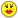+0

# 2 is 4% of?

#2
+8

Good try, selena......!!!

Let's look at this again

"is" means " = "       "of" means "multiply"        and 4%   = .04        so we have

2 = .04 N      divide both sides by .04

2 / .04 = N

50 = NDec 11, 2014

#1
+3

hopefully im right but i think its 2/0.4 which equals 5 ok

With regards

SelenaDec 11, 2014
#2
+8

Good try, selena......!!!

Let's look at this again

"is" means " = "       "of" means "multiply"        and 4%   = .04        so we have

2 = .04 N      divide both sides by .04

2 / .04 = N

50 = NCPhill Dec 11, 2014